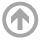Mathematics Department

# Combinatorics, Algebra, & Topology Seminar

## Fall 2017

All talks are from 1200-1300 in the designated room unless otherwise specified.

• Dec
04
• MIDN Research Presentations
USNA
Time: 12:00 PM
• Dec
01
• Schur positivity in a special case of the Delta Conjecture
Emily Sergel
University of Pennsylvania
Time: 12:00 PM

#### View Abstract

The modified Macdonald polynomials are an important basis for the ring of symmetric functions with two parameters, q and t. There are several open problems regarding the combinatorics of these polynomials. For example, they are known to expand positively into the Schur basis, but the coefficients of this expansion are unknown. The Shuffle Conjecture was another famous example, but it was proved in 2015 after being open for over a decade. We discuss a related and still-open conjecture called the Delta Conjecture. The Delta conjecture equates a certain symmetric function with a weighted sum of combinatorial objects called parking functions. The combinatorial side of this conjecture can be expressed in terms of LLT polynomials, which implies Schur positivity. However, Schur positivity on the symmetric function side is unclear. After a detailed introduction to the world of Macdonald polynomials and parking functions, I will prove that, in a special case, the coefficient of any Schur function on the symmetric function side of the Delta conjecture is not just positive, but a positive sum of q,t-analogs. My talk will assume no prior knowledge of symmetric function theory. (Joint work with Dun Qui, Jeff Remmel and Guoce Xin.)
• Nov
27
• The combinatorics of symmetric quotient rings
Andrew Wilson
University of Pennsylvania
Time: 12:00 PM

#### View Abstract

he coinvariant ring of the symmetric group is the quotient of the polynomial ring by the ideal generated by all symmetric polynomials without a constant term. Many properties of this ring are closely connected to the combinatorics of the symmetric group. What if, instead, we mod out by an ideal generated by some other set of polynomials? If the ideal is symmetric, can we use combinatorics to understand the properties of the resulting quotient ring? A variety of authors (Rhoades, Haglund, Shimozono, Huang, Scrimshaw, the speaker, and others) have discovered many well-behaved quotient rings this way. Furthermore, they have shown that the rings are connected to classical combinatorial objects like ordered set partitions and words. We will provide an overview of the work in this area and pose a conjecture that, if proven, would unify much of the existing work on this problem.
• Nov
09
• Michael Tait
Carnegie Mellon University
Time: 12:00 PM

#### View Abstract

How many edges may be in an n-vertex graph that does not contain a triangle? Can a set of integers "look like" both an arithmetic progression and a geometric progression at the same time? These are examples of questions in extremal graph theory and combinatorial number theory respectively. In this talk, we discuss how to use finite incidence geometries (eg, a projective plane) to prove theorems in these areas of combinatorics.
• Nov
06
• Degree Sequence Packing
James Shook
National Institute of Standards and Technology
Time: 12:00 PM
• Nov
02
• A stable arithmetic regularity lemma in finite-dimensional vector spaces over fields of prime order
Caroline Terry
University of Maryland
Time: 12:00 PM

#### View Abstract

In this talk we present a stable version of the arithmetic regularity lemma for vector spaces over fields of prime order. The arithmetic regularity lemma for $\mathbb{F}_p^n$ (first proved by Green in 2005) states that given $A\subseteq \F_p^n$, there exists $H\leq \F_p^n$ of bounded index such that $A$ is Fourier-uniform with respect to almost all costs of $H$. In general, the growth of the index of $H$ is required to be of tower type depending on the degree of uniformity, and must also allow for a small number of non-uniform elements. Our main result is that, under a natural stability theoretic assumption, the bad bounds and non-uniform elements are not necessary. Specifically, we present an arithmetic regularity lemma for k-stable sets $A\subseteq \mathbb{F}_p^n$, where the bound on the index of the subspace is only polynomial in the degree of uniformity, and where there are no non-uniform elements. This result is a natural extension to the arithmetic setting of the work on stable graph regularity lemmas initiated by Malliaris and Shelah. This is joint work with Julia Wolf.
• Oct
30
• Franklin Kenter
USNA
Time: 12:00 PM
• Oct
16
• David Joyner
USNA
Time: 12:00 PM
• Sep
11
• Grothendieck polynomials and the technique of iterated residues
Justin Allman
USNA
Time: 12:00 PM

#### View Abstract

The stable Grothendieck polynomials are symmetric functions which are a non-homogeneous deformation of Schur functions. In fact, they are the representatives of the Schubert varieties in the K-theory of the Grassmannian (a role played by Schur functions in cohomology). Recently, Richard Rimanyi and I have shown that the technique of iterated residues can be used to explain many of their important properties; in particular, I will discuss "straightening laws" and the multiplication of stable Grothendieck polynomials in this context. One interesting special case of the multiplicative structure is the K-theoretic Pieri rule, for which we give a new (to our knowledge) formulation.

#### ARCHIVES